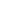A can do a work in 10 days and B in 20 days. If they together work on it for 5 days, then the fraction of the work that is left is:

a) 4/3
b) 1/4
c) 3/4
d) 3/20
SSC CGL-2015

 1/4. Solution A's 1 day work = 1/10 B's 1 day work = 1/20 (A+B)'s 1 day work = 1/10 + 1/20 = 3/20 (A+B)'s 5 day work = 5 x 3/20 = 15/20 Fraction of work left = 5/20 = 1/4
Share :
+
Next
« Prev
Prev
Next »